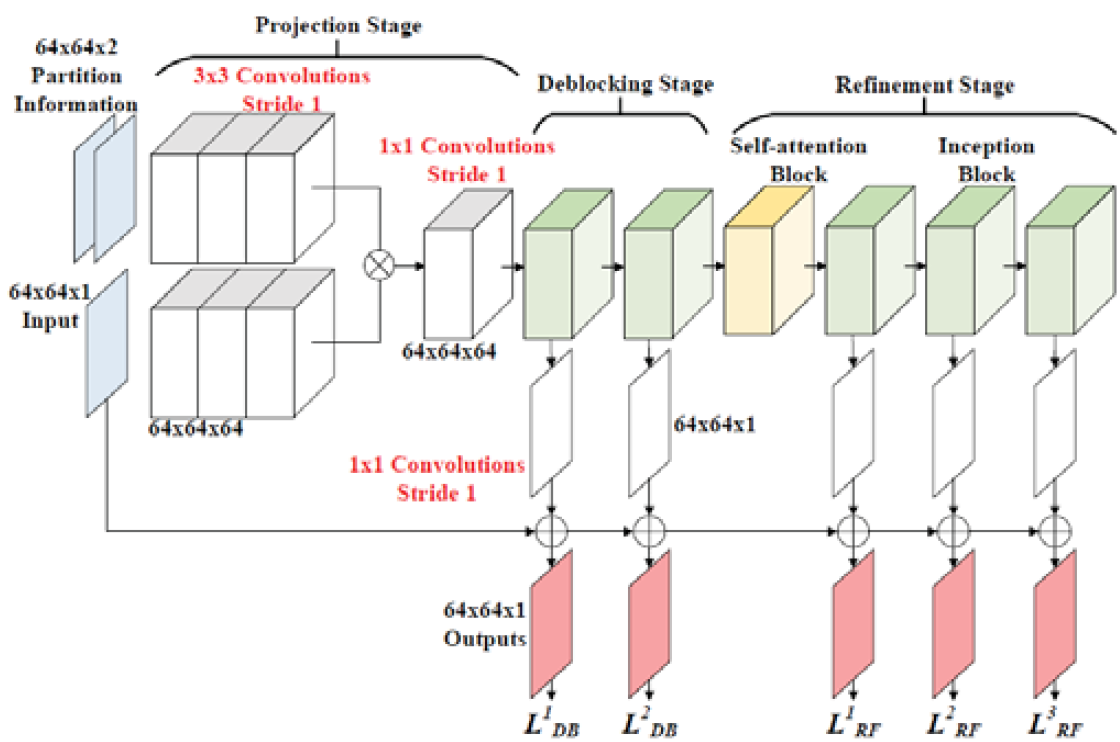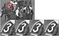# Reading: MACNN — Multi-stage Attention Convolutional Neural Network for HEVC (Codec Filtering)

## Outperforms VRCNN, DCAD, MMS-net, VDSR, DS-CNN, MPRGAN

this story, Multi-stage Attention Convolutional Neural Network (MACNN), by National Tsing Hua University, is presented. In this paper, an in-loop or post processing filter is proposed:

• Revised Inception block is used.
• Self-Attention block is utilized.
• A new loss function is introduced.

This is a paper in 2020 AICAS. This conference is launched since 2019 which is a quite new conference, which is about AI for Circuits and Systems. The conference is held in August this year (2020), yet the accepted papers can be previewed right now. (Sik-Ho Tsang @ Medium)

# Outline

1. MACNN Network Architecture
2. Loss Function
3. Experimental Results

# 1. MACNN Network ArchitectureMACNN Network Architecture (This figure in the paper also needs to be filtered…lol)
• There are 3 stages: First, coded images and partition information are projected to feature space then merged.
• Next, the merged feature map is exploited to attenuate blocking artifacts.
• Finally, long-range dependencies are captured and utilized to reduce general artifacts and recover details as well.

## 1.1. Projection Stage

• First, a raw CTU is normalized to 0 and 1 beforehand as input.
• The CU partition and TU partition information is converted into two 2-dimensional maps as input, in which ‘1’ denotes the borders of CU and TU while ‘0’ denotes the others.
• After pre-processing, the inputs are sent to convolutional layers. All the layers apply 3×3 filters with stride 1, generating 64 feature maps.
• When it comes to feature fusion, concatenation and 1×1 convolution are usually taken into account. 1×1 convolution is able to learn how to shrink the numbers of feature maps while preserving the entire information.

## 1.2. Deblocking Stage

• The 3×3 convolution branch and the 5×5 convolution branch are preserved while the max-pooling branch and the 1×1 convolution branch are discarded for simplicity, as shown above.
• The activation function is also replaced with PReLU.
• Each output from blocks at the stage will be sent to 1×1 convolution layers and the next block simultaneously.
• 1×1 convolution layers exploit the denoised feature maps for reconstruction while deeper blocks utilized it for further feature extraction and denoising.
• The combination of mean square error and the partition error we proposed as loss function at the deblocking stage, which will be mentioned in more details later.

## 1.3. Refinement Stage

• The Refinement stage adopts a Self-attention Block and three revised Inception Blocks for general artifacts reduction and further refinement
• Self-attention Block is able to model the correlation between any two positions of the input feature maps regardless of their spatial distance.
• The ⨂ denotes matrix multiplication. The softmax operation is performed on each row.
• At the upper path, with the transpose and matrix multiplication, long-range dependencies can be learnt.

# 2. Loss Function

• There are two kinds of losses, one at deblocking stage and one at refinement stage.

## 2.1. Loss Function at Deblocking Stage

• Standard MSE loss is used:
• Also, partition loss is used as well:
• where PCU and PTU denote the CU partition maps and the TU partition maps, ⊙ denotes element-wise multiplication.
• And the loss at deblocking stage is the weighted sum of the above two losses:
• where j means the j-th output block at the deblocking stage.

## 2.2. Loss Function at Refinement Stage

• To eliminate general artifacts such as ringing and blurring, Sobel loss is used where G is sobel operator:
• Integrating L1-gradient into loss may help optimization algorithms get rid of being stuck in local minima and focus on high frequency structures.
• And the loss at refinement stage is the weighted sum of the MSE loss and Sobel loss:
• Finally, the total loss is LDB+LRF.

# 3. Experimental Results

• The MACNN is compared with two kinds of models, namely models for HEVC in-loop filters replacement and models for post-processing only.
• “All Intra Main” configuration is used with five QP values, including 22, 27, 32, 37 and 42.
• While training, a CPIH-Intra database, proposed by Li ICME’17 is used.
• The models for QP=37 and QP=42 are trained from scratch, others are fine-tuned.
• MACNN obtains 6.5% BD-rate reduction which outperforms VRCNN and DCAD.
• MACNN outperforms MMS-net (M=5) a bit, but inferior to MMS-net (M=15).
• The reason is that with relatively larger (30) number of residual blocks, thus the number of parameters in MMS-net (M=15) is approximately three times as many as MACNN one, which as shown above.
• By comparing PSNR, MACNN is 45.0% higher than VRCNN, 24.6% higher than DS-CNN, and 7.0% higher than MPRGAN.Visual Quality at QP=37 (Original, Before Filtering, After HEVC Filtering, After MACNN)
• As shown above, it is obvious that by using MACNN, various artifacts such as blocking (such as upper-left corner), ringing (contour of character “3”), and blurring are attenuated while sharp edges are preserved.

During the days of coronavirus, A challenge of writing 30/35/40 stories again for this month has been accomplished. Let me challenge 45 stories!! This is the 43rd story in this month.. Thanks for visiting my story..

## Reference

[2020 AICAS] [MACNN]
Multi-stage Attention Convolutional Neural Networks for HEVC In-Loop Filtering

## Codec Filtering

JPEG [ARCNN] [RED-Net] [DnCNN] [Li ICME’17] [MemNet] [MWCNN]
HEVC [Lin DCC’16] [IFCNN] [VRCNN] [DCAD] [MMS-net] [DRN] [Lee ICCE’18] [DS-CNN] [RHCNN] [VRCNN-ext] [S-CNN & C-CNN] [MLSDRN] [Double-Input CNN] [B-DRRN] [Residual-VRN] [Liu PCS’19] [QE-CNN] [EDCNN] [VRCNN-BN] [MACNN]
3D-HEVC [RSVE+POST]
AVS3 [Lin PCS’19]
VVC
[Lu CVPRW’19] [Wang APSIPA ASC’19]